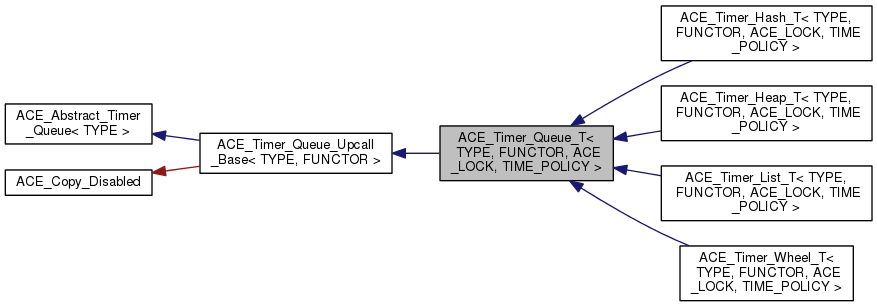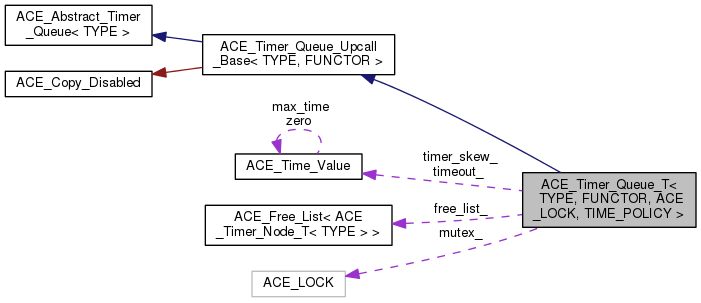ACE  6.1.2
ACE_Timer_Queue_T< TYPE, FUNCTOR, ACE_LOCK, TIME_POLICY > Class Template Reference

Provides an interface to timers. More...

`#include <Timer_Queue_T.h>`

Inheritance diagram for ACE_Timer_Queue_T< TYPE, FUNCTOR, ACE_LOCK, TIME_POLICY >:[legend]
Collaboration diagram for ACE_Timer_Queue_T< TYPE, FUNCTOR, ACE_LOCK, TIME_POLICY >:[legend]

List of all members.

## Public Member Functions

ACE_Timer_Queue_T (FUNCTOR *upcall_functor=0, ACE_Free_List< ACE_Timer_Node_T< TYPE > > *freelist=0, TIME_POLICY const &time_policy=TIME_POLICY())
virtual ~ACE_Timer_Queue_T (void)
virtual long schedule (const TYPE &type, const void *act, const ACE_Time_Value &future_time, const ACE_Time_Value &interval=ACE_Time_Value::zero)
virtual int dispatch_info (const ACE_Time_Value &current_time, ACE_Timer_Node_Dispatch_Info_T< TYPE > &info)
virtual void gettimeofday (ACE_Time_Value(*gettimeofday)(void))
ACE_Time_Value gettimeofday_static ()
void set_time_policy (TIME_POLICY const &time_policy)
void timer_skew (const ACE_Time_Value &skew)
Set the timer skew for the Timer_Queue.
const ACE_Time_Valuetimer_skew (void) const
Get the timer skew for the Timer_Queue.
ACE_LOCK & mutex (void)
Synchronization variable used by the queue.
virtual void dump (void) const
Dump the state of a object.
virtual void return_node (ACE_Timer_Node_T< TYPE > *)
void preinvoke (ACE_Timer_Node_Dispatch_Info_T< TYPE > &info, const ACE_Time_Value &cur_time, const void *&upcall_act)
This method will call the preinvoke() on <functor>.
void upcall (ACE_Timer_Node_Dispatch_Info_T< TYPE > &info, const ACE_Time_Value &cur_time)
This method will call the timeout() on <functor>.
void postinvoke (ACE_Timer_Node_Dispatch_Info_T< TYPE > &info, const ACE_Time_Value &cur_time, const void *upcall_act)
This method will call the postinvoke() on <functor>.
virtual int expire (const ACE_Time_Value &current_time)
virtual int expire (void)
virtual int expire_single (ACE_Command_Base &pre_dispatch_command)
virtual ACE_Time_Value gettimeofday (void)
virtual ACE_Time_Valuecalculate_timeout (ACE_Time_Value *max)
virtual ACE_Time_Valuecalculate_timeout (ACE_Time_Value *max, ACE_Time_Value *the_timeout)
virtual ACE_Time_Value current_time ()

## Protected Member Functions

virtual long schedule_i (const TYPE &type, const void *act, const ACE_Time_Value &future_time, const ACE_Time_Value &interval)=0
Schedule a timer.
virtual void reschedule (ACE_Timer_Node_T< TYPE > *)=0
Reschedule an "interval" ACE_Timer_Node.
virtual ACE_Timer_Node_T< TYPE > * alloc_node (void)
Factory method that allocates a new node.
virtual void free_node (ACE_Timer_Node_T< TYPE > *)
Factory method that frees a previously allocated node.
virtual int dispatch_info_i (const ACE_Time_Value &current_time, ACE_Timer_Node_Dispatch_Info_T< TYPE > &info)
Non-locking version of dispatch_info ()
void recompute_next_abs_interval_time (ACE_Timer_Node_T< TYPE > *expired, const ACE_Time_Value &cur_time)
Recompute when the next time is that this interval timer should fire.

## Protected Attributes

ACE_LOCK mutex_
ACE_Free_List
< ACE_Timer_Node_T< TYPE > > *
free_list_
Class that implements a free list.
TIME_POLICY time_policy_
The policy to return the current time of day.
bool const delete_free_list_
Flag to delete only if the class created the <free_list_>

## Private Attributes

ACE_Time_Value timeout_
Returned by <calculate_timeout>.
ACE_Time_Value timer_skew_
Adjusts for timer skew in various clocks.

## Detailed Description

### template<class TYPE, class FUNCTOR, class ACE_LOCK, typename TIME_POLICY = ACE_Default_Time_Policy> class ACE_Timer_Queue_T< TYPE, FUNCTOR, ACE_LOCK, TIME_POLICY >

Provides an interface to timers.

This is an abstract base class that provides hook for implementing specialized policies such as ACE_Timer_List and ACE_Timer_Heap.

## Constructor & Destructor Documentation

template<class TYPE, class FUNCTOR, class ACE_LOCK , typename TIME_POLICY>
 ACE_Timer_Queue_T< TYPE, FUNCTOR, ACE_LOCK, TIME_POLICY >::ACE_Timer_Queue_T ( FUNCTOR * upcall_functor = `0`, ACE_Free_List< ACE_Timer_Node_T< TYPE > > * freelist = `0`, TIME_POLICY const & time_policy = `TIME_POLICY()` )

Default constructor. upcall_functor is the instance of the FUNCTOR to be used by the queue. If upcall_functor is 0, Timer Queue will create a default FUNCTOR. freelist the freelist of timer nodes. If 0, then a default freelist will be created.

template<class TYPE , class FUNCTOR , class ACE_LOCK , typename TIME_POLICY >
 ACE_Timer_Queue_T< TYPE, FUNCTOR, ACE_LOCK, TIME_POLICY >::~ACE_Timer_Queue_T ( void )
virtual

Destructor - make virtual for proper destruction of inherited classes.

## Member Function Documentation

template<class TYPE , class FUNCTOR , class ACE_LOCK , typename TIME_POLICY >
 ACE_Timer_Node_T< TYPE > * ACE_Timer_Queue_T< TYPE, FUNCTOR, ACE_LOCK, TIME_POLICY >::alloc_node ( void )
protectedvirtual

Factory method that allocates a new node.

Reimplemented in ACE_Timer_Heap_T< TYPE, FUNCTOR, ACE_LOCK, TIME_POLICY >.

template<class TYPE , class FUNCTOR , class ACE_LOCK , typename TIME_POLICY >
 ACE_Time_Value * ACE_Timer_Queue_T< TYPE, FUNCTOR, ACE_LOCK, TIME_POLICY >::calculate_timeout ( ACE_Time_Value * max )
virtual

Determine the next event to timeout. Returns max if there are no pending timers or if all pending timers are longer than max. This method acquires a lock internally since it modifies internal state. Implement calculate_timeout() using the right locking policy

Implements ACE_Abstract_Timer_Queue< TYPE >.

template<class TYPE , class FUNCTOR , class ACE_LOCK , typename TIME_POLICY >
 ACE_Time_Value * ACE_Timer_Queue_T< TYPE, FUNCTOR, ACE_LOCK, TIME_POLICY >::calculate_timeout ( ACE_Time_Value * max, ACE_Time_Value * the_timeout )
virtual

Determine the next event to timeout. Returns max if there are no pending timers or if all pending timers are longer than max. This method acquires a lock internally since it modifies internal state. Implement calculate_timeout() using the right locking policy

Implements ACE_Abstract_Timer_Queue< TYPE >.

template<class TYPE , class FUNCTOR , class ACE_LOCK , typename TIME_POLICY >
 ACE_Time_Value ACE_Timer_Queue_T< TYPE, FUNCTOR, ACE_LOCK, TIME_POLICY >::current_time ( )
virtual

Determine the next event to timeout. Returns max if there are no pending timers or if all pending timers are longer than max. This method acquires a lock internally since it modifies internal state. Implement calculate_timeout() using the right locking policy

Implements ACE_Abstract_Timer_Queue< TYPE >.

template<class TYPE, class FUNCTOR , class ACE_LOCK , typename TIME_POLICY >
 int ACE_Timer_Queue_T< TYPE, FUNCTOR, ACE_LOCK, TIME_POLICY >::dispatch_info ( const ACE_Time_Value & current_time, ACE_Timer_Node_Dispatch_Info_T< TYPE > & info )
virtual

Get the dispatch information for a timer whose value is <= current_time. This does not account for <timer_skew>. Returns 1 if there is a node whose value <= current_time else returns a 0.

template<class TYPE, class FUNCTOR , class ACE_LOCK , typename TIME_POLICY >
 int ACE_Timer_Queue_T< TYPE, FUNCTOR, ACE_LOCK, TIME_POLICY >::dispatch_info_i ( const ACE_Time_Value & current_time, ACE_Timer_Node_Dispatch_Info_T< TYPE > & info )
protectedvirtual

Non-locking version of dispatch_info ()

Reimplemented in ACE_Timer_Hash_T< TYPE, FUNCTOR, ACE_LOCK, BUCKET, TIME_POLICY >.

template<class TYPE , class FUNCTOR , class ACE_LOCK , typename TIME_POLICY >
 void ACE_Timer_Queue_T< TYPE, FUNCTOR, ACE_LOCK, TIME_POLICY >::dump ( void ) const
virtual

Dump the state of a object.

Implements ACE_Abstract_Timer_Queue< TYPE >.

template<class TYPE , class FUNCTOR , class ACE_LOCK , typename TIME_POLICY >
 int ACE_Timer_Queue_T< TYPE, FUNCTOR, ACE_LOCK, TIME_POLICY >::expire ( const ACE_Time_Value & current_time )
virtual

Implement ACE_Abstract_Timer_Queue<TYPE>::expire () with the right locking strategy.

Implements ACE_Abstract_Timer_Queue< TYPE >.

template<class TYPE , class FUNCTOR , class ACE_LOCK , typename TIME_POLICY >
 int ACE_Timer_Queue_T< TYPE, FUNCTOR, ACE_LOCK, TIME_POLICY >::expire ( void )
inlinevirtual

Implement ACE_Abstract_Timer_Queue<TYPE>::expire () with the right locking strategy.

Implements ACE_Abstract_Timer_Queue< TYPE >.

template<class TYPE , class FUNCTOR , class ACE_LOCK , typename TIME_POLICY >
 int ACE_Timer_Queue_T< TYPE, FUNCTOR, ACE_LOCK, TIME_POLICY >::expire_single ( ACE_Command_Base & pre_dispatch_command )
virtual

Implement ACE_Abstract_Timer_Queue<TYPE>::expire () with the right locking strategy.

Implements ACE_Abstract_Timer_Queue< TYPE >.

template<class TYPE, class FUNCTOR , class ACE_LOCK , typename TIME_POLICY >
 void ACE_Timer_Queue_T< TYPE, FUNCTOR, ACE_LOCK, TIME_POLICY >::free_node ( ACE_Timer_Node_T< TYPE > * node )
protectedvirtual

Factory method that frees a previously allocated node.

template<class TYPE , class FUNCTOR , class ACE_LOCK , typename TIME_POLICY >
 ACE_Time_Value ACE_Timer_Queue_T< TYPE, FUNCTOR, ACE_LOCK, TIME_POLICY >::gettimeofday ( void )
virtual

Implement the gettimeofday() virtual function

Implements ACE_Abstract_Timer_Queue< TYPE >.

template<class TYPE , class FUNCTOR , class ACE_LOCK , typename TIME_POLICY >
 void ACE_Timer_Queue_T< TYPE, FUNCTOR, ACE_LOCK, TIME_POLICY >::gettimeofday ( ACE_Time_Value(*)(void) gettimeofday )
virtual

Allows applications to control how the timer queue gets the time of day.

Deprecated:
Use TIME_POLICY support instead. This will only have effect when the TIME_POLICY used is ACE_FPointer_Time_Policy. Other standard ACE time policies will ignore this.

Implements ACE_Abstract_Timer_Queue< TYPE >.

template<class TYPE , class FUNCTOR , class ACE_LOCK , typename TIME_POLICY >
 ACE_Time_Value ACE_Timer_Queue_T< TYPE, FUNCTOR, ACE_LOCK, TIME_POLICY >::gettimeofday_static ( void )
inline

Implement an inlined, non-abstract version of gettimeofday(), through this member function the internals of the class can make calls to ACE_OS::gettimeofday() with zero overhead.

template<class TYPE , class FUNCTOR , class ACE_LOCK , typename TIME_POLICY >
 ACE_LOCK & ACE_Timer_Queue_T< TYPE, FUNCTOR, ACE_LOCK, TIME_POLICY >::mutex ( void )

Synchronization variable used by the queue.

template<class TYPE, class FUNCTOR , class ACE_LOCK , typename TIME_POLICY >
 void ACE_Timer_Queue_T< TYPE, FUNCTOR, ACE_LOCK, TIME_POLICY >::postinvoke ( ACE_Timer_Node_Dispatch_Info_T< TYPE > & info, const ACE_Time_Value & cur_time, const void * upcall_act )
inline

This method will call the postinvoke() on <functor>.

template<class TYPE, class FUNCTOR , class ACE_LOCK , typename TIME_POLICY >
 void ACE_Timer_Queue_T< TYPE, FUNCTOR, ACE_LOCK, TIME_POLICY >::preinvoke ( ACE_Timer_Node_Dispatch_Info_T< TYPE > & info, const ACE_Time_Value & cur_time, const void *& upcall_act )
inline

This method will call the preinvoke() on <functor>.

template<class TYPE, class FUNCTOR , class ACE_LOCK , typename TIME_POLICY >
 void ACE_Timer_Queue_T< TYPE, FUNCTOR, ACE_LOCK, TIME_POLICY >::recompute_next_abs_interval_time ( ACE_Timer_Node_T< TYPE > * expired, const ACE_Time_Value & cur_time )
protected

Recompute when the next time is that this interval timer should fire.

template<class TYPE, class FUNCTOR, class ACE_LOCK, typename TIME_POLICY = ACE_Default_Time_Policy>
 virtual void ACE_Timer_Queue_T< TYPE, FUNCTOR, ACE_LOCK, TIME_POLICY >::reschedule ( ACE_Timer_Node_T< TYPE > * )
protectedpure virtual

Reschedule an "interval" ACE_Timer_Node.

template<class TYPE, class FUNCTOR , class ACE_LOCK , typename TIME_POLICY >
 void ACE_Timer_Queue_T< TYPE, FUNCTOR, ACE_LOCK, TIME_POLICY >::return_node ( ACE_Timer_Node_T< TYPE > * node )
virtual

Method used to return a timer node to the queue's ownership after it is returned by a method like <remove_first>.

template<class TYPE, class FUNCTOR , class ACE_LOCK , typename TIME_POLICY >
 long ACE_Timer_Queue_T< TYPE, FUNCTOR, ACE_LOCK, TIME_POLICY >::schedule ( const TYPE & type, const void * act, const ACE_Time_Value & future_time, const ACE_Time_Value & interval = `ACE_Time_Value::zero` )
virtual

Implement ACE_Abstract_Timer_Queue<TYPE>::schedule () with the right locking strategy.

Implements ACE_Abstract_Timer_Queue< TYPE >.

template<class TYPE, class FUNCTOR, class ACE_LOCK, typename TIME_POLICY = ACE_Default_Time_Policy>
 virtual long ACE_Timer_Queue_T< TYPE, FUNCTOR, ACE_LOCK, TIME_POLICY >::schedule_i ( const TYPE & type, const void * act, const ACE_Time_Value & future_time, const ACE_Time_Value & interval )
protectedpure virtual
template<class TYPE , class FUNCTOR , class ACE_LOCK , typename TIME_POLICY>
 void ACE_Timer_Queue_T< TYPE, FUNCTOR, ACE_LOCK, TIME_POLICY >::set_time_policy ( TIME_POLICY const & time_policy )
inline

Allows applications to control how the timer queue gets the time of day.

template<class TYPE , class FUNCTOR , class ACE_LOCK , typename TIME_POLICY >
 void ACE_Timer_Queue_T< TYPE, FUNCTOR, ACE_LOCK, TIME_POLICY >::timer_skew ( const ACE_Time_Value & skew )
inline

Set the timer skew for the Timer_Queue.

template<class TYPE , class FUNCTOR , class ACE_LOCK , typename TIME_POLICY >
 const ACE_Time_Value & ACE_Timer_Queue_T< TYPE, FUNCTOR, ACE_LOCK, TIME_POLICY >::timer_skew ( void ) const
inline

Get the timer skew for the Timer_Queue.

template<class TYPE, class FUNCTOR , class ACE_LOCK , typename TIME_POLICY >
 void ACE_Timer_Queue_T< TYPE, FUNCTOR, ACE_LOCK, TIME_POLICY >::upcall ( ACE_Timer_Node_Dispatch_Info_T< TYPE > & info, const ACE_Time_Value & cur_time )
inline

This method will call the timeout() on <functor>.

## Member Data Documentation

template<class TYPE, class FUNCTOR, class ACE_LOCK, typename TIME_POLICY = ACE_Default_Time_Policy>
 bool const ACE_Timer_Queue_T< TYPE, FUNCTOR, ACE_LOCK, TIME_POLICY >::delete_free_list_
protected

Flag to delete only if the class created the <free_list_>

template<class TYPE, class FUNCTOR, class ACE_LOCK, typename TIME_POLICY = ACE_Default_Time_Policy>
 ACE_Free_List >* ACE_Timer_Queue_T< TYPE, FUNCTOR, ACE_LOCK, TIME_POLICY >::free_list_
protected

Class that implements a free list.

template<class TYPE, class FUNCTOR, class ACE_LOCK, typename TIME_POLICY = ACE_Default_Time_Policy>
 ACE_LOCK ACE_Timer_Queue_T< TYPE, FUNCTOR, ACE_LOCK, TIME_POLICY >::mutex_
protected

Synchronization variable for ACE_Timer_Queue.

Note:
The right name would be lock_, but HP/C++ will choke on that!
template<class TYPE, class FUNCTOR, class ACE_LOCK, typename TIME_POLICY = ACE_Default_Time_Policy>
 TIME_POLICY ACE_Timer_Queue_T< TYPE, FUNCTOR, ACE_LOCK, TIME_POLICY >::time_policy_
protected

The policy to return the current time of day.

template<class TYPE, class FUNCTOR, class ACE_LOCK, typename TIME_POLICY = ACE_Default_Time_Policy>
 ACE_Time_Value ACE_Timer_Queue_T< TYPE, FUNCTOR, ACE_LOCK, TIME_POLICY >::timeout_
private

Returned by <calculate_timeout>.

template<class TYPE, class FUNCTOR, class ACE_LOCK, typename TIME_POLICY = ACE_Default_Time_Policy>
 ACE_Time_Value ACE_Timer_Queue_T< TYPE, FUNCTOR, ACE_LOCK, TIME_POLICY >::timer_skew_
private

Adjusts for timer skew in various clocks.

The documentation for this class was generated from the following files: